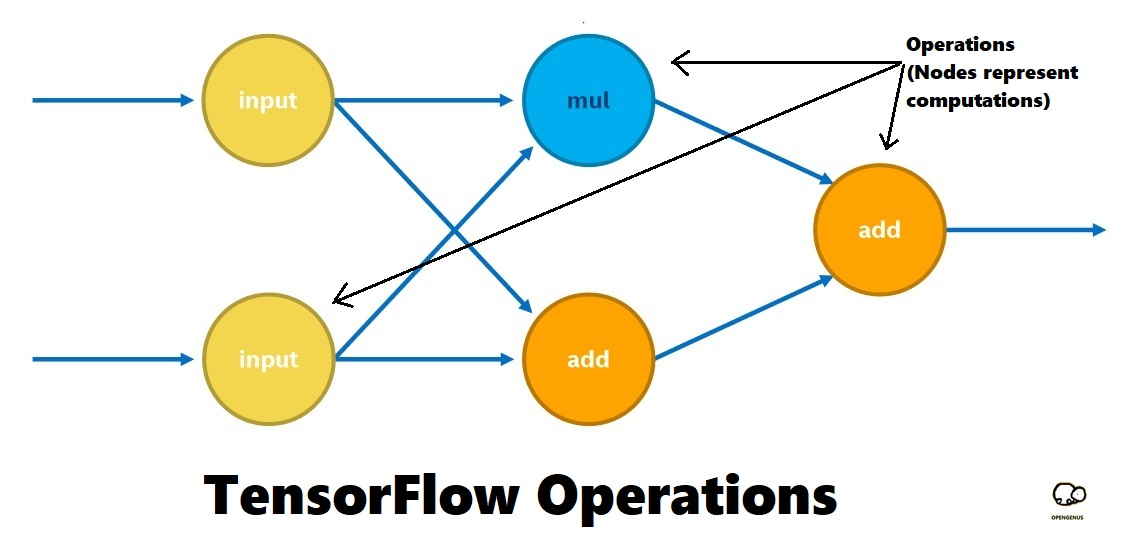Get FREE domain for 1st year and build your brand new siteIn this article, we have implemented a TensorFlow code in Python to add two numbers with tf.add() and have explained the basic concepts involved like Placeholder, operation and session. We have demonstrated how to optimize the code as well.

1. TF code to add 2 numbers
2. Placeholder
3. TF Operation
4. Session
2. Optimize the TensorFlow code

# TF code to add 2 numbers

The TensorFlow code in Python to add 2 numbers is as follows:

``````import tensorflow as tf
# Create a placeholder data a
a = tf.placeholder(tf.float32, name="input1")
# Create a placeholder data b
b = tf.placeholder(tf.float32, name="input2")
# Create an add operation using a and b
sess = tf.Session()
feed_dict = {a: 3.0, b: 2.0}
output = sess.run(c, feed_dict)
print (output)
``````

The concepts involved into this simple code are:

1. Placeholder
2. TF Operation
3. Session

### 1. Placeholder

To define an user defined data, use:

• tf.placeholder() defines an user defined input that vary run to run

In general Machine Learning applications, we have a process known as training which generates some of the input parameters such as weights and bias to be used. For it, we need to use tf.Variable()

### 2. TF Operation

Operations are nodes in the TensorFlow Computational Graph and it represents mathematical operations to be computed. The number of inputs and outputs depends on the operation represented by the operation node.

Some of the common operation nodes are:

• Multiplication
• Matrix Multiplication
• Convolution

and others### 3. Session

A TensorFlow session is like a runtime or a container where the program will execute. To create a session, use:

``````sess = tf.Session()
``````

To execute the graph, use:

``````sess.run(graph, feed_dict)
``````

sess.run returns the fetched values as a NumPy array.

# Optimize the TensorFlow code

To optimize the code, we can see configuration parameters like inter threads and intra threads in the session. This allows better use of threads and parallel execution.

Intra threads mean threads to run the operation in a parallel way.

A session has several properties and to set it, we need ConfigProto. It is used to set configurations of the Session object.

This code creates a session and sets two custom configuration values:

``````config = tf.ConfigProto( inter_op_parallelism_threads=1,
tf.Session(config=config)
``````

The optimized code for adding two numbers in TensorFlow is as follows:

``````import tensorflow as tf
# Create a placeholder data a
a = tf.placeholder(tf.float32, name="input1")
# Create a placeholder data b
b = tf.placeholder(tf.float32, name="input2")
# Create an add operation using a and b
sess = tf.Session(config=config)
feed_dict = {a: 3.0, b: 2.0}
output = sess.run(c, feed_dict)
print (output)
``````

In addition to this, you can do the following:

• Set OMP_NUM_THREADS in the terminal using:
``````export OMP_NUM_THREADS = 128
``````

The number should be equal to the number of cores in the system.

• Use TCMalloc

To use TCMalloc, build it and set the LD_LIBRARY_PATH enironment variable. This will optimize memory allocation calls in TensorFlow.

Optimizations may not bring much improvement in this simple example of adding two numbers but in larger code, it becomes critical.

With this article at OpenGenus, you must have a strong idea of writing a TensorFlow code in Python to add 2 numbers. Try to multiply two numbers using TensorFlow.